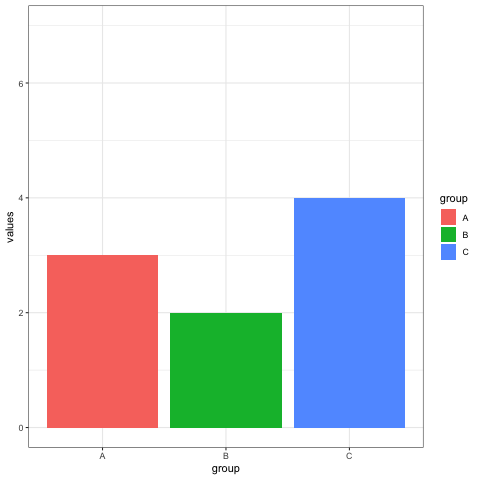# Animated barplot transition with R

The `gganimate` package allows to build animated chart using the ggplot2 syntax directly from R. This post describes how to make an animation between 2 barplot states.

# Smooth barplot transition

Before trying to build an animated plot with `gganimate`, make sure you understood how to build a basic bar chart with R and `ggplot2`.

The idea is to add an additional aesthetics called `transition_..()` that provides a frame variable. For each value of the variable, a step on the chart will be drawn. Here, `transition_states()` is used since the frame variable is categorical.

Note that the `gganimate` automatically performs a transition between state. Several options are available, set using the `ease_aes()` function.``````# libraries:
library(ggplot2)
library(gganimate)

# Make 2 basic states and concatenate them:
a <- data.frame(group=c("A","B","C"), values=c(3,2,4), frame=rep('a',3))
b <- data.frame(group=c("A","B","C"), values=c(5,3,7), frame=rep('b',3))
data <- rbind(a,b)

# Basic barplot:
ggplot(a, aes(x=group, y=values, fill=group)) +
geom_bar(stat='identity')

# Make a ggplot, but add frame=year: one image per year
ggplot(data, aes(x=group, y=values, fill=group)) +
geom_bar(stat='identity') +
theme_bw() +
# gganimate specific bits:
transition_states(
frame,
transition_length = 2,
state_length = 1
) +
ease_aes('sine-in-out')

# Save at gif:
anim_save("288-animated-barplot-transition.gif")``````

Related chart types

## Contact

This document is a work by Yan Holtz. Any feedback is highly encouraged. You can fill an issue on Github, drop me a message on Twitter, or send an email pasting yan.holtz.data with gmail.com.